# Olympiad Test: Geometrical Shapes And Angles - 2

## 10 Questions MCQ Test National Cyber Olympiad Class 5 | Olympiad Test: Geometrical Shapes And Angles - 2

Description
Attempt Olympiad Test: Geometrical Shapes And Angles - 2 | 10 questions in 20 minutes | Mock test for Class 5 preparation | Free important questions MCQ to study National Cyber Olympiad Class 5 for Class 5 Exam | Download free PDF with solutions
QUESTION: 1

### Which one of the following angles is obtuse?

Solution:

Half a right angle = 45°
One right angle = 90°
One and a half right angles = 1½ × 90° = 135°
Two right angles = 180°
Only 135° is greater than 90° but less than 180°, so it is the only obtuse angle.

QUESTION: 2

### How many straight angles are there in three full rotations?

Solution:

A full rotation consists of two straight (180°) angles. So three full rotations consist of six straight angles.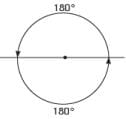QUESTION: 3

### Which one of the following angles is not reflex?

Solution:

A reflex angle is more than 180° but less than 360°. 178° is less than 180°, so it is not reflex. (All the rest are reflex angles.)

QUESTION: 4

Which one of the following angles is reflex?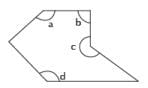Solution:

A Reflex Angle is greater than 180° but less than 360°. A, B and D are all less than 180°.  Only C is greater than 180° but less than 360°.

QUESTION: 5

If angle AOB = 67°, what is the size of reflex angle AOB?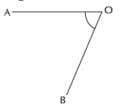Solution:

If you add the smaller angle (acute or obtuse) and the reflex angle for the same shape you will always come to 360°. Therefore, reflex angle AOB = 360° − 67° = 293°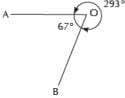QUESTION: 6

What is the least number of 33° angles you would need to make a reflex angle?

Solution:

2 × 33° = 66°, which is between 0° and 90°, so acute.
5 × 33° = 165°, which is between 90° and 180°, so obtuse.
6 × 33° = 198°, which is between 180° and 360°, so reflex.
7 × 33° = 231°, which is also reflex, but we have already found the least amount which is 6.

QUESTION: 7

Which of the following describes the triangle shown above?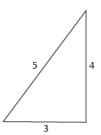Solution:

The triangle has three different lengths of side, so is scalene. The triangle has lengths 3, 4 and 5 and 52 = 32 + 42, so is right angled. Therefore, it is a scalene right angled triangle.

QUESTION: 8

A triangle is isosceles and right angled. Which of the following statements must be false?

Solution:

Since the triangle is isosceles, it must have two equal angles. Since it is also right angled, the other two angles must both be 45° (45° + 45° +90° = 180°). So A is true.
Since the triangle is isosceles, it must have two of its sides equal.
So B is true.
An isosceles triangle has one line of symmetry.
So C is also true.
A triangle that satisfies A, B and C is shown in the following diagram: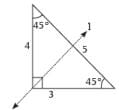Line l is the axis of symmetry
Statement D must be false since a triangle with three different sides cannot be isosceles.

QUESTION: 9

Shraddha made two copies of the right angled triangle shown and cut them out.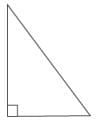Q. She then joined edges of the triangles together to make shapes.  Which of the following shapes was it not possible for Shraddha to make?

Solution:

Shraddha could make an isosceles triangle, a parallelogram, a rectangle or a kite: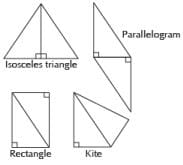But the rectangle is not a square because the sides have different lengths.

QUESTION: 10

Shraddha made two copies of the following isosceles right angled triangle and cut them out. She then joined edges of the triangles together to make shapes.

Q. Which of the following shapes was it NOT possible for Shraddha to make?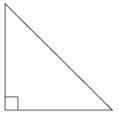Solution:

Shraddha could make a square, an isosceles triangle (one with 2 equal sides, 2 equal angles), and a parallelogram: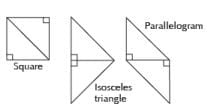But she could not make an equilateral triangle (one with all equal sides, all equal angles).Use Code STAYHOME200 and get INR 200 additional OFF Use Coupon Code